Next: Twisted Symmetry Groups Up: Symmetries of Columns Previous: Classification of Subgroups of

## Untwisted Symmetry Groups

Suppose that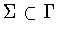is a symmetry group. Then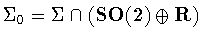is one of the subgroups listed in Table  1. We say that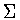is an untwisted subgroup ofifis conjugate to a subgroup of the formwhere K is contained in the subgroup H given in Table  1. The untwisted symmetry groups are listed in Table  2.It is not the case that every subgroup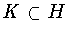produces a symmetry group. For example, when,the only symmetry groupcorresponding tois.(This isindependent of the restriction to untwisted symmetry groups.) To verify this point, observe that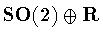acts transitively on the cylinder.Hence ifis the symmetry group of a function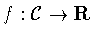,then f is the constant function.It follows that f is invariant under,and that the symmetrysubgroup.

Whencontains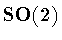,the function f isconstant on each horizontal cross-section ofand hence automaticallyhas the symmetry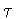.In these cases, the only possibilities areand.Similarly, whencontainsthen automaticallyand the only possibilities areand.

In all other cases, there are no restrictions on K other than thecondition.Next: Twisted Symmetry Groups Up: Symmetries of Columns Previous: Classification of Subgroups of
Marty Golubitsky
2001-01-29